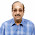1. What is the number of zeros at the end of the product of the numbers from 1 to 100?

2. A fast typist can type some matter in 2 hours and a slow typist can type the same in 3 hours. If both type combinely, in how much time will they finish?

3. Gavaskar's average in his first 50 innings was 50. After the 51st innings, his average was 51. How many runs did he score in his 51st innings. (supposing that he lost his wicket in his 51st innings)

4. A rectangular plate with length 8 inches, breadth 11 inches and thickness 2 inches is available. What is the length of the circular rod with diameter 8 inches and equal to the volume of the rectangular plate?

5. What is the sum of all numbers between 100 and 1000 which are divisible by 14 ?

6. If s(a) denotes square root of a, find the value of s(12+s(12+s(12+ ...... upto infinity.

7. A cylindrical container has a radius of eight inches with a height of three inches. Compute how many inches should be added to either the radius or height to give the same increase in volume?

8. With just six weights and a balance scale, you can weigh any unit number of kgs from 1 to 364. What could be the six weights?

9. Diophantus passed one sixth of his life in childhood, one twelfth in youth, and one seventh more as a bachelor; five years after his marriage a son was born who died four years before his father at half his final age. How old is Diophantus

10 . If time at this moment is 9 P.M., what will be the time 23999999992 hours later?

1. 127

2. 1 hr 12 min Explanation : The fast typist's work done in 1 hr = 1/2 The slow typist's work done in 1 hr = 1/3 If they work combinely, work done in 1 hr = 1/2+1/3 = 5/6 So, the work will be completed in 6/5 hours. i.e., 1+1/5 hours = 1hr 12 min

3. 101 Explanation : Total score after 50 innings = 50*50 = 2500 Total score after 51 innings = 51*51 = 2601 So, runs made in the 51st innings = 2601-2500 = 101 If he had not lost his wicket in his 51st innings, he would have scored an unbeaten 50 in his 51st innings.

4. 3.5 inches Explanation : Volume of the circular rod (cylinder) = Volume of the rectangular plate (22/7)*4*4*h = 8*11*2 h = 7/2 = 3.5

5. 35392 Explanation : The number closest to 100 which is greater than 100 and divisible by 14 is 112, which is the first term of the series which has to be summed. The number closest to 1000 which is less than 1000 and divisible by 14 is 994, which is the last term of the series. 112 + 126 + .... + 994 = 14(8+9+ ... + 71) = 35392

6. 4 , Explanation : Let x = s(12+s(12+s(12+..... We can write x = s(12+x). i.e., x^2 = 12 + x. Solving this quadratic equation, we get x = -3 or x=4. Sum cannot be -ve and hence sum = 4.

7. 16/3 inches Explanation : Let x be the amount of increase. The volume will increase by the same amount if the radius increased or the height is increased. So, the effect on increasing height is equal to the effect on increasing the radius. i.e., (22/7)*8*8*(3+x) = (22/7)*(8+x)*(8+x)*3 Solving the quadratic equation we get the x = 0 or 16/3. The possible increase would be by 16/3 inches.

8. 1, 3, 9, 27, 81, 243 (All powers of 3)

9. 84 years Explanation : x/6 + x/12 + x/7 + 5 + x/2 + 4 = x

10 . 1 P.M. Explanation : 24 billion hours later, it would be 9 P.M. and 8 hours before that it would be 1 P.M.

1 comment:

1.NICELY posted. very useful questions.
thanks
sambasivan